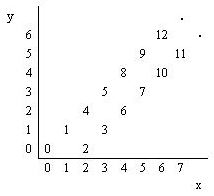Online JudgeProblem SetAuthorsOnline ContestsUser
Web Board
F.A.Qs
Statistical Charts
Problems
Submit Problem
Online Status
Prob.ID:
Register
Authors ranklist
Current Contest
Past Contests
Scheduled Contests
Award Contest
Register
Language:
Number Steps
 Time Limit: 1000MS Memory Limit: 10000K Total Submissions: 15059 Accepted: 8039

Description

Starting from point (0,0) on a plane, we have written all non-negative integers 0,1,2, ... as shown in the figure. For example, 1, 2, and 3 has been written at points (1,1), (2,0), and (3, 1) respectively and this pattern has continued.You are to write a program that reads the coordinates of a point (x, y), and writes the number (if any) that has been written at that point. (x, y) coordinates in the input are in the range 0...5000.

Input

The first line of the input is N, the number of test cases for this problem. In each of the N following lines, there is x, and y representing the coordinates (x, y) of a point.

Output

For each point in the input, write the number written at that point or write No Number if there is none.

Sample Input

```3
4 2
6 6
3 4```

Sample Output

```6
12
No Number```

Source

[Submit]   [Go Back]   [Status]   [Discuss]Home PageGo BackTo top